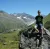Crownnote is where music fans share their favorite songs.

Chart

# 2021 End Of The Year - Top 50

1. Number
1
2. Number
2
3. Number
3
4. Number
4
5. Number
5
6. Number
6
7. Number
7
8. Number
8
9. Number
9
10. Number
10
11. Number
11
12. Number
12
13. Number
13
14. Number
14
15. Number
15
16. Number
16
17. Number
17
18. Number
18
19. Number
19
20. Number
20
21. Number
21
22. Number
22
23. Number
23
24. Number
24
25. Number
25
26. Number
26
27. Number
27
28. Number
28
29. Number
29
30. Number
30
31. Number
31
32. Number
32
33. Number
33
34. Number
34
35. Number
35
36. Number
36
37. Number
37
38. Number
38
39. Number
39
40. Number
40
41. Number
41
42. Number
42
43. Number
43
44. Number
44
45. Number
45
46. Number
46
47. Number
47
48. Number
48
49. Number
49
50. Number
50
51. Dropouts
1. Number
3 Dropout
Peak
3
Weeks
4
2. Number
8 Dropout
Peak
8
Weeks
3
3. Number
9 Dropout
Peak
9
Weeks
1
4. Number
10 Dropout
Peak
9
Weeks
10
5. Number
12 Dropout
Peak
12
Weeks
2
6. Number
14 Dropout
Peak
14
Weeks
1
7. Number
15 Dropout
Peak
15
Weeks
1
8. Number
16 Dropout
Peak
15
Weeks
8
9. Number
17 Dropout
Peak
13
Weeks
6
10. Number
18 Dropout
Peak
18
Weeks
3

A chart byRuisdier
Date
Songs
50
No points